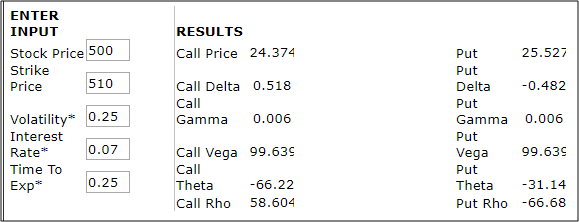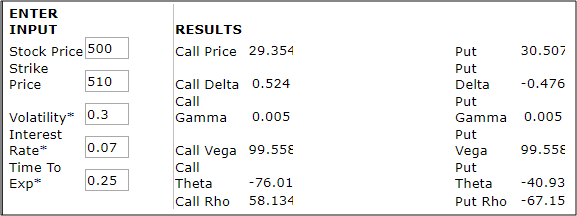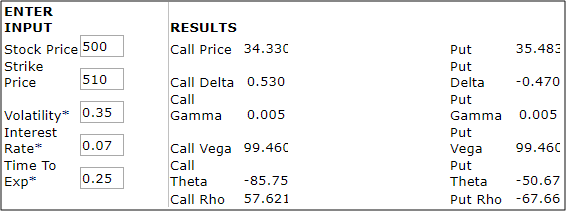Why does volatility impact call and put options in the same way - Motilal Oswal
Why does volatility impact call and put options in the same way - Motilal Oswal

# Why does volatility impact call and put options in the same way?What do we understand by the term volatility? Simply put, volatility is a measure of risk. But what exactly is volatility in technical terms? It can be seen as the standard deviation of returns from the mean. Every investor wants an investment that is able to give predictable returns over a period of time. When returns become too volatile they become unpredictable. That is the time when the asset becomes less valuable. Normally stocks that are too volatile are not preferred by investors and they command a lower valuation. But did you know that the reverse applies in case of options. In fact, volatility positively impacts the values of call options and put options.

Normally, volatility and asset prices are inversely related. Higher the volatility, higher is the risk and when the perceived risk is high lower are the returns compared to expectations. Investors are always more willing to pay for safety rather than for volatility. But when it comes to call and put options, the scenario is entirely different. Calls and put options actually gain in value when the volatility in the market increases. So, let us understand why option prices increase with volatility. Let us also understand the relationship between option volatility and pricing. How does that impact the implied volatility call vs put? Let us look at the impact on call options first..

Volatility and their impact on call and put options
What do we understand by volatility? It means that the returns on the stock are likely to be volatile around the mean. It also means that the level of uncertainty is too high in this case. But why does that positively impact the value of the put option? Let us get back to the basic Black & Scholes model first..
According to the Black Scholes model, there are 5 key factors that impact the price of an option as under:

Market Price of the Stock: An increase in the stock price positively impacts the call option value but negatively impacts the put option value

Strike Price of the Stock: A shift to higher strike prices reduces the value of a call option but increases the value of a put option

Interest rates: An increase in the interest rates reduces the present value of the strike price and makes the call option more valuable and the put option less valuable

An reduction in the time to maturity or time to expiry reduces the value of a call option and also the put option

An increase in the volatility of the stock increases the value of the call options and also of the put option.
As can be seen from the above points, it is only volatility that impacts call and put options in the same direction. The case is the same for time to expiry but that is a subset of volatility since longer time to expiry builds greater expectations of volatility. But why is it that volatility impacts calls and puts in the same direction.

The reasons are not far to seek. An option (both calls and puts) are non-symmetric. That means, the buyer of the option will only exercise the option when it is favourable and choose to forego the premium when the price movement is negative. This rule applies to call options and to put options. Higher volatility means higher upside risk or higher downside risk. When there is downside risk, the buyer of the call option will forego the premium. When there is upside risk, the buyer of the call option will rake in the profits. The same rule applies to put options too. That is why higher volatility makes call options and put options more valuable.

Understanding volatility and options price will real examples
To understand this point from a better perspective, we have considered the same option (call and put) under different levels of volatility. In scenario 1 we assume volatility at 25%..In the above case we can see that when the options are near the money, the delta of the call and the put are around the half-way mark. We have assumed that all the other factors will remain constant through… Now let us look at the situation when the volatility increases to 30%.When the volatility goes up from 25% to 30% we can see that the value of the call and put option have gone up simultaneously as per the Black & Scholes model. Now let also look at a scenario wherein the volatility goes up further to 35%..As can be seen the price of the call and put are going up further due to higher volatility. This clearly explains that an increase in volatility positively impacts the value of the call option and the put option, other factors remaining constant!